# Carleson measure

(diff) ← Older revision | Latest revision (diff) | Newer revision → (diff)
Jump to: navigation, search

Carleson measures were introduced in the early 1960s by L. Carleson [a1] to characterize the interpolating sequences in the algebraof bounded analytic functions in the open unit disc and to give a solution to the corona problem (cf. also Hardy spaces).

These measures can be defined in the following way: Letbe a positive measure on the unit disc. Thenis called a Carleson measure if there exists a constantsuch that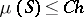for every sector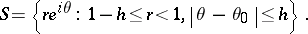Carleson measures play an important role in complex analysis (cf. also Analytic function), harmonic analysis,theory (cf. also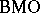-space), the theory of integral operators, and the theory of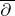-equations (cf. also Neumann-problem). One of Carleson's original theorems states that, with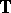denoting the boundary of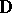, for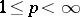the Poisson operator (cf. also Poisson integral)is a bounded linear operator from the Hardy space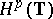to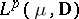if and only ifis a Carleson measure. Generalizations of this principle to various other function spaces in one or several real or complex variables have been given. Carleson measures and their generalizations can also be used to give complete characterizations of boundedness and compactness of composition operators on various spaces of analytic functions, such as Hardy and Bergman spaces (see [a2]).